# 数据结构和算法

## 数据结构

### 三、链表

#### 1. 单向链表

``````class ListNode:
def __init__(self, x):
self.val = x
self.next = None
``````

#### 2. 双向链表

``````class ListNode:
def __init__(self, x):
self.val = x
self.next = None
self.prev = None
``````

#### 4. 双向循环链表(1) 链表是链式的存储结构；数组是顺序的存储结构。
(2) 链表通过指针来连接元素与元素；数组则是把所有元素按次序依次存储。
(3) 链表的插入删除元素相对数组较为简单，不需要移动元素，且较为容易实现长度扩充，但是寻找某个元素较为困难。
(4) 数组寻找某个元素较为简单，但插入与删除比较复杂，由于最大长度需要再编程一开始时指定，故当达到最大长度时，扩充长度不如链表方便。

### 四、字典和散列表

#### 2. 散列表（HashMap）

(1) 冲突版
HashMap数组每一个元素的初始值都是Null。调用 hashMap.put("apple", 0)，插入一个key为“apple”的元素。这时候我们需要利用一个哈希函数来确定Entry的插入位置：

``````index = Hash('apple')
``````因为HashMap的长度是有限的，当插入的Entry越来越多时，再完美的Hash函数也难免会出现index冲突的情况。

(2) 链表
HashMap数组的每一个元素不止是一个Entry对象，也是一个链表的头节点。每一个Entry对象通过Next指针指向它的下一个Entry节点。当新来的Entry映射到冲突的数组位置时，只需要插入到对应的链表即可。(3) 线性探查

### 五、二叉树

#### 1. 常见二叉树

(1) 二叉搜索树

(2) 二叉平衡树

``````class Solution:
def IsBalanced_Solution(self, root):
if not root:
return True
if abs(self.maxDepth(root.left) - self.maxDepth(root.right)) > 1:
return False
return self.IsBalanced_Solution(root.left) and self.IsBalanced_Solution(root.right)

def maxDepth(self, root):
if not root: return 0
return max(self.maxDepth(root.left), self.maxDepth(root.right)) + 1
``````

(3) 堆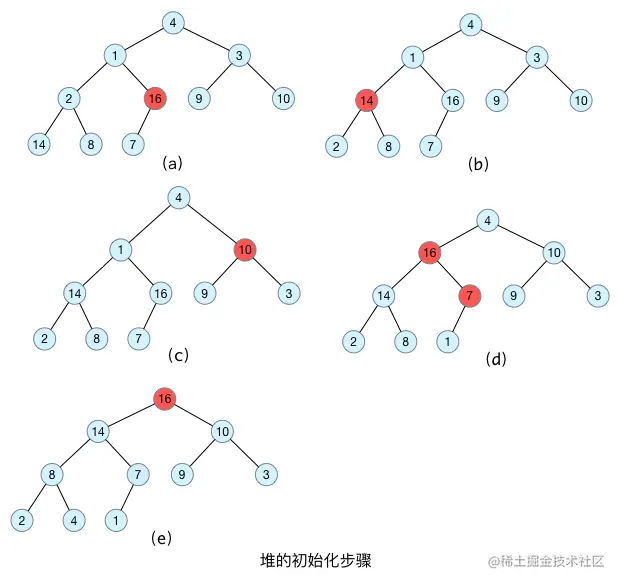#### 2. 二叉树遍历

(1) 中序遍历：左根右

``````res = []
def dfs(root):
if not root: return
dfs(root.left)
res.append(root)
dfs(root.right)
``````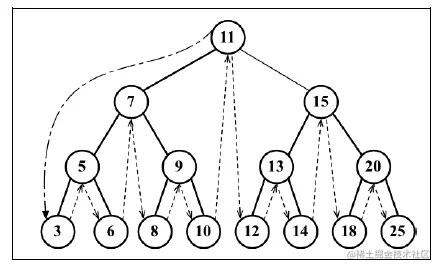3-5-6-7-8-9-10-11-12-13-14-15-18-20-25

(2) 先序遍历：根左右

``````res = []
def dfs(root):
if not root:return
res.append(root)
dfs(root.left)
dfs(root.right)
``````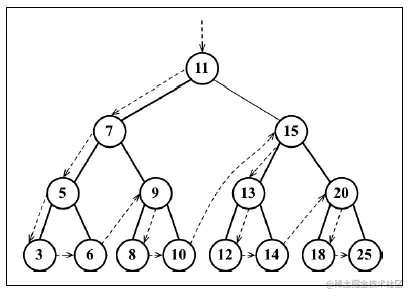11-7-5-3-6-9-8-10-15-13-12-14-20-18-25

(3) 后序遍历：左右根

``````res = []
def dfs(root):
if not root:return
res.append(root)
dfs(root.left)
dfs(root.right)
``````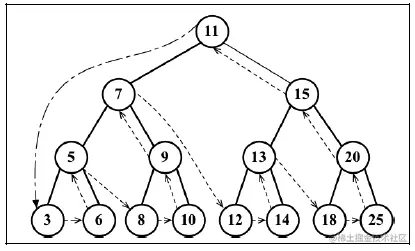3-6-5-8-10-9-7-12-14-13-18-25-20-15-11

### 六、排序算法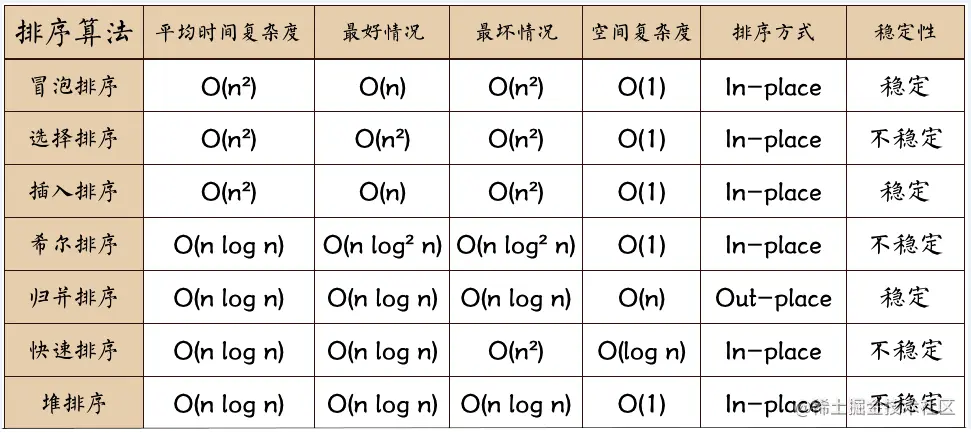#### 1. 冒泡排序

(1) 比较相邻的两个元素，如果前一个比后一个大，则交换位置。
(2) 第一轮的时候最后一个元素应该是最大的一个。
(3) 按照步骤一的方法进行相邻两个元素的比较，这个时候由于最后一个元素已经是最大的了，所以最后一个元素不用比较。

``````def bubble_sort(list):
for i in range(len(list)-1,0,-1):
for j in range(i):
if list[j] > list[j+1]:
list[j],list[j+1] = list[j+1],list[j]
return list
``````

#### 2. 选择排序

(1) 在未排序序列中找到最小元素，存放到排序序列的起始位置。
(2) 再从剩余未排序元素中继续寻找最小元素，然后放到已排序序列的末尾。

``````def select_sort(list):
length = len(list)
for i in range(length):
min = i
for j in range(i,length):
if list[j] < list[min]:
min = j
list[i],list[min] = list[min],list[i]
return list
``````

#### 3. 插入排序

(1) 假设数列第一个元素为已排序数列，剩余数列为未排序。
(2) 将待排序元素挨个插入到已排序数列中每次插入都必须保证数列是有序的。

``````def insert_sort(list):
length = len(list)
for i in range(1,length):
for j in range(i):
if list[j] > list[j+1]:
list[j],list[j+1] = list[j+1],list[j]
return list
``````

#### 4. 希尔排序

(1) 将整个待排序的列分割成为若干子序列。
(2) 分别进行直接插入排序。

``````def shell_sort(slist):
gap = len(slist)
while gap > 1:
gap = gap // 2
for i in range(gap, len(slist)):
for j in range(i % gap, i, gap):
if slist[i] < slist[j]:
slist[i], slist[j] = slist[j], slist[i]
return slist
``````

#### 5. 归并排序

``````def merge_sort(array):
def merge_arr(arr_l, arr_r):
array = []
while len(arr_l) and len(arr_r):
if arr_l <= arr_r:
array.append(arr_l.pop(0))
elif arr_l > arr_r:
array.append(arr_r.pop(0))
if len(arr_l) != 0:
array += arr_l
elif len(arr_r) != 0:
array += arr_r
return array
``````

#### 6. 快速排序

``````def quick_sort(list):
if list == []:
return []
else:
first = list
left = quick_sort([l for l in list[1:]if l < first])
right = quick_sort([l for l in list[1:] if l > first])
return left +[first] + right
``````

#### 7. 堆排序

``````def heap_sort(array):
child = 2 * parent + 1  # left child
while child < len(heap):
if child + 1 < len(heap):
if heap[child + 1] > heap[child]:
child += 1  # right child
if heap[parent] >= heap[child]:
break
heap[parent], heap[child] = \
heap[child], heap[parent]
parent, child = child, 2 * child + 1

heap, array = array.copy(), []
for i in range(len(heap) // 2, -1, -1):
while len(heap) != 0:
heap, heap[-1] = heap[-1], heap
array.insert(0, heap.pop())
return array
``````

### 七、LeetCode相关

#### 1. Python

1 数字

``````import math
#向上取整
math.ceil(2.1)

#向下取整
math.floor(2.9)

#四舍五入
def round(n):
return math.ceil(n) if (n - math.floor(n)) >= 0.5 else math.floor(n)
``````

2 字符串

api作用
len()计算长度
capitalize(),lower(), upper()大小写
center(s), ljust(s), rjust(s)补全
count(s)出现次数
find(s),lfind(s),rfind(s)查找位置
split()分割成列表
replace(old, new [, max])替换字符串
strip(), lstrip(), rstrip()去空格
``````#center(s), ljust(s), rjust(s)
'aaa'.center('b') #'baaab'

#capitalize(), lower(), upper()
'AAA'.lower() #'aaa'

#count()
'aaa'.count('a') #3

#find()
'abc'.find('a') #0

#split()
'a b c'.split() #['a','b','c']
``````

3 列表

api作用
len()计算长度
append(x),insert(I,x),pop(i),remove(x)增删
sort(reverse=False)排序
reverse()反转列表
max(),min()最大最小
count()计数
extend(l)列表合并
index()查找
``````#append(x), insert(i,x), pop(i), remove(x)
[1,2,3].append(4) #[1,2,3,4]
[1,2,3].insert(1,1.5) #[1, 1.5, 2, 3]
[1,2,3].pop(1) #[1,3]
['a','b','c'].remove('b') #['a', 'c']

#sort(reverse=False)
[1,2,3].sort(reverse=True) #[3,2,1]

#reverse()
[1,2,3].reverse() #[3,2,1]

#index
[1,2,3].index(2) #1

#count
[1,2,3].count(2) #1

#join
''.join(['a','b','c']) #abc
``````

``````def f(x):
return x * x
map(f, [1, 2, 3]) #[1,4,9]

from functools import reduce
return x + y

def is_odd(n):
return n % 2 == 1
filter(is_odd, [1, 2, 4]) // 
``````

4 字典

api作用
dict.keys()取键
dict.values()取值

#### 2. JavaScript

1 Array 数组常用方法

``````let a = [1, 2, 3]
a.splice(1, 1, 4, 5) // 
a // [1, 4, 5, 3]
["a", "b", "c"].slice(1,2)  // ["b"]

["a", "b", "c"].join('-')   // "a-b-c"
["a", "b", "c"].concat("d")   // ["a", "b", "c", "d"]

[3,1,2].sort((a,b) => a-b)  // [1, 2, 3]
["a", "b", "c"].reverse()   // ["c", "b", "a"]

push(), pop(), unshift(), shift()

[3, 10, 18, 20].some(val => val>18)   // true
[3, 10, 18, 20].every(val => val>18)    // false
[3, 10, 18, 20].filter(val => val>18)   // 

[1,2,3].forEach(val => val+1)   // undefined
[1,2,3].map(val => val+1)  // [2, 3, 4]

arr.reduce(callback,[initialValue])
[1, 2, 3].reduce(function(prev, cur, index, arr) {
console.log(prev, cur, index, arr);
return prev + cur;
},1)  // 7

[1,2,3].indexOf(2)  // 1
``````

2 String 字符串常用方法

``````"hello".indexOf('h')  // 0
"hello".match("e")  // ["e", index: 1, input: "hello", groups: undefined]   String.match(regexp)
"hello".search("h") //0   String.search(regexp)

"hello".charAt(1)   // "e"
"hello".concat("world")   // "helloworld"
"hello".replace("o","ooo")  // "hellooo"

"abc".slice(1,2)  // "b"
"abc".split("") // ["a", "b", "c"]
"hello".substr(2,3) // "llo"
"hellow".substring(2,3) // "l"

toLowerCase(), toUpperCase()
``````

3 Object

``````Object.keys({a:1,b:2})  // ["a", "b"]
Object.values({a:1,b:2})  // [1, 2]
``````

#### 3. 进制转换

3.1 十进制转二进制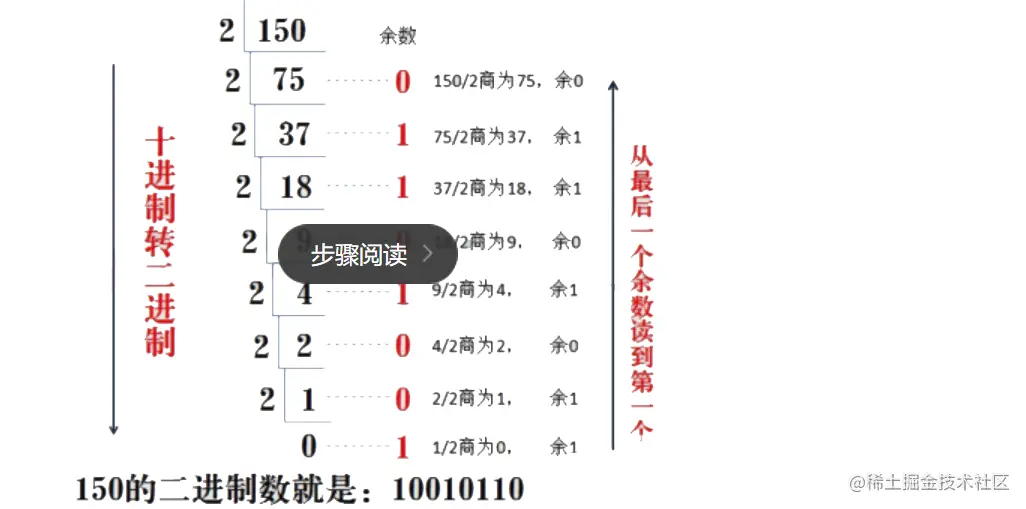3.2 二进制转十进制

3.3 二进制转八进制
3位二进制数按权展开相加得到1位八进制数。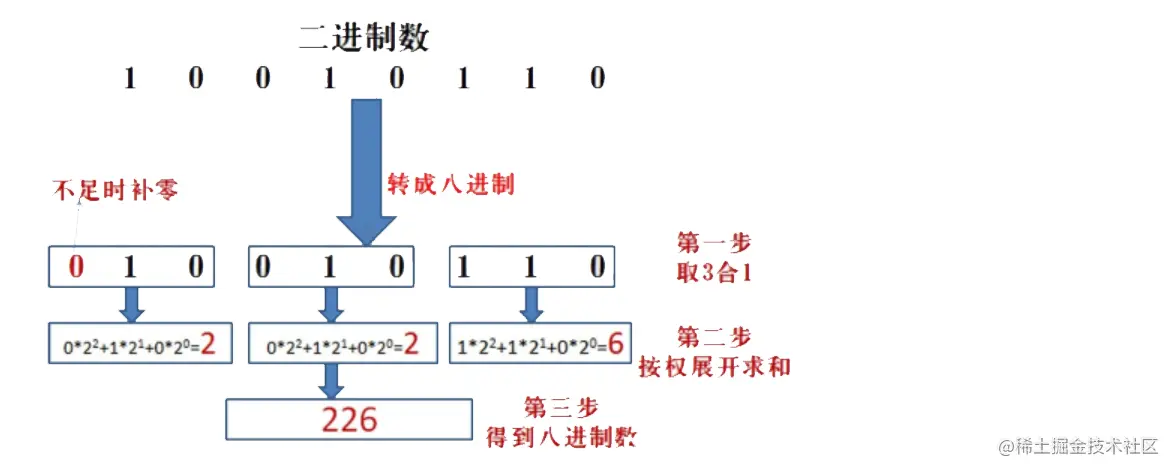3.4 八进制转成二进制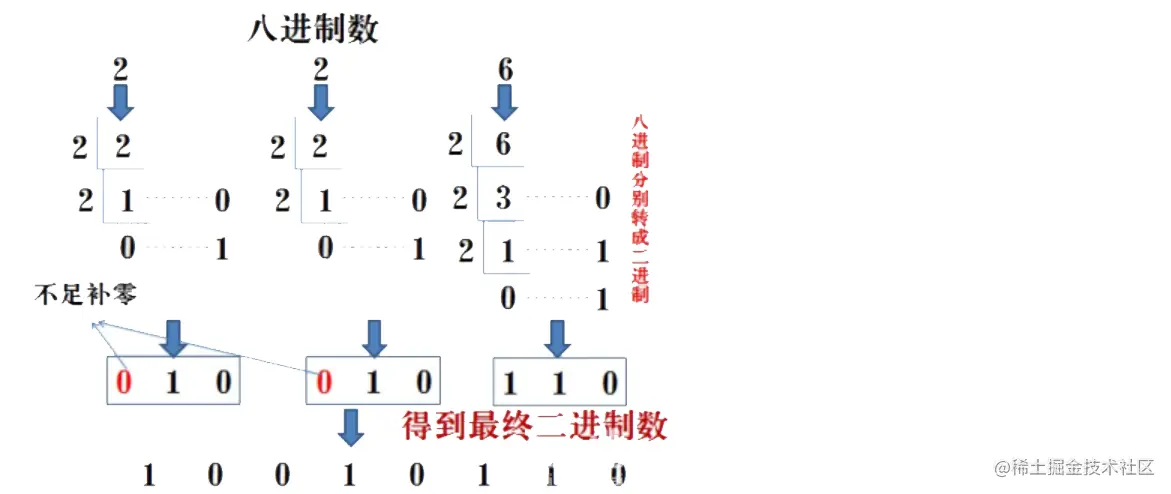3.5 二进制转十六进制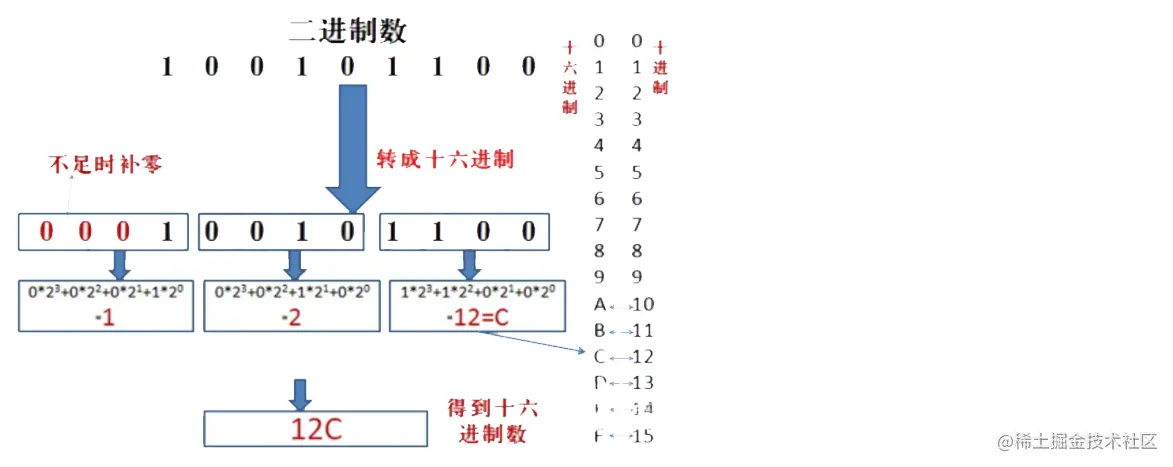3.6 十六进制转二进制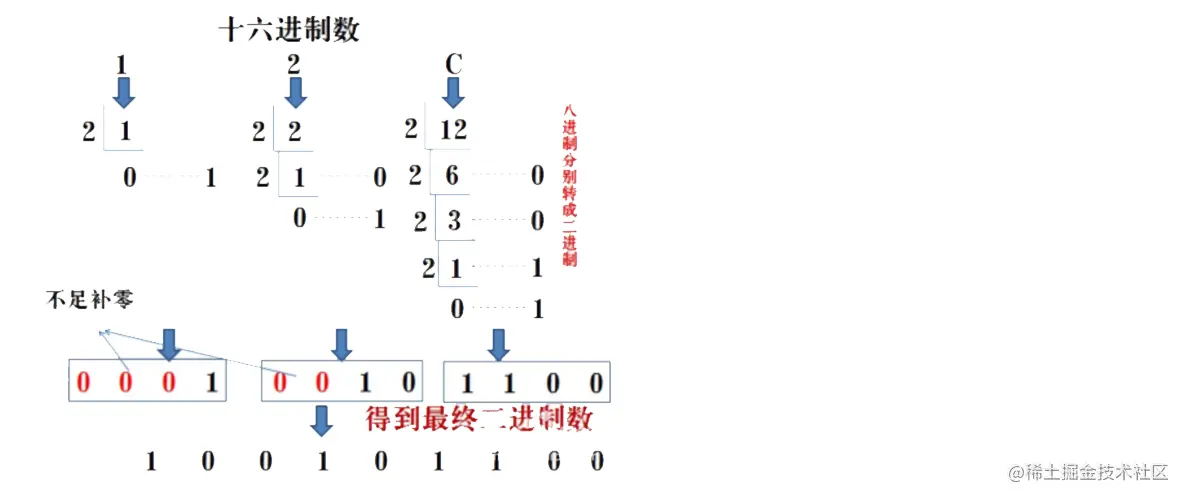3.7 十进制转八进制或者十六进制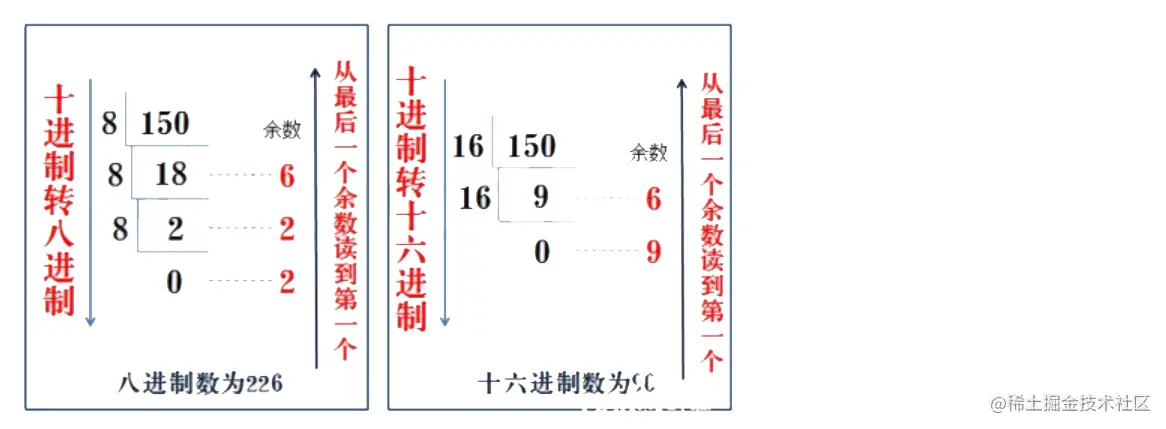3.8 八进制或者十六进制转成十进制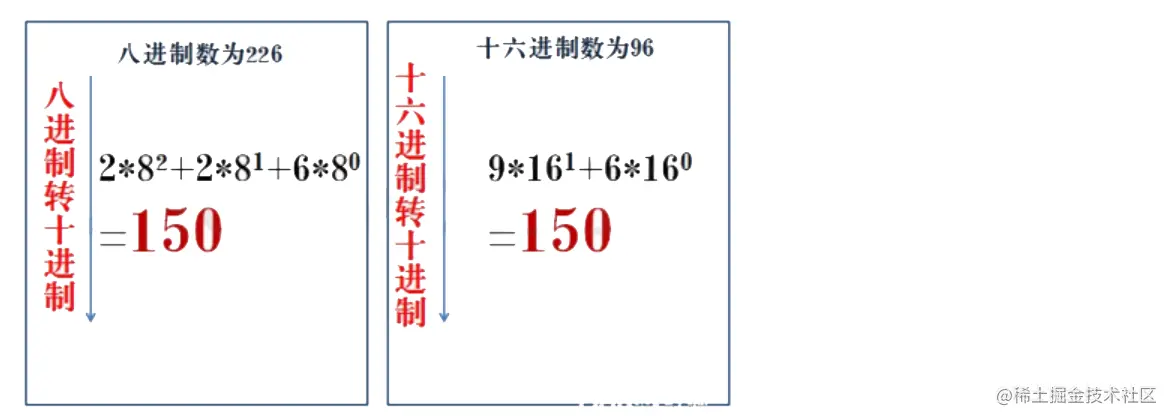3.9 api

JavaScript

``````// 其他 to 十进制
// 十进制 to 其他
``````

Python

``````bin(x, radix) // 2进制
``````

#### 4. 位操作

4.1 位操作

&两个位都为1时，结果才为1
|两个位都为0时，结果才为0
^异或两个位相同为0，相异为1
~取反0变1，1变0
<<左移各二进位全部左移若干位，高位丢弃，低位补0
>>右移各二进位全部右移若干位，对无符号数，高位补0，有符号数，各编译器处理方法不一样，有的补符号位（算术右移），有的补0（逻辑右移）

4.2 位操作常见场景

``````sum1 = num1^num2 // 异或代表两数相加但不进位
sum2 = (num1&num2)<<1 // 进位值
return sum1 + sum2
``````

``````// 数 a 向右移一位，相当于将 a 除以 2；数 a 向左移一位，相当于将 a 乘以 2
a = 2
a >> 1 // 1
a << 1 //4
``````

``````// 只要根据数的最后一位是 0 还是 1 来决定即可
a & 1 == 0
``````

``````a ^= b
b ^= a
a ^= b
``````

``````// 整数取反加1，正好变成其对应的负数(补码表示)；负数取反加一，则变为其原码，即正数
~a + 1
``````

``````// 正数右移 31 位得到 0，负数右移 31 位得到 -1
sign = a >> 31;
i == 0 ? a : (~a + 1)
``````

``````// 每计算一次二进制中就少了一个 1
count = 0
while(a){
a = a & (a - 1)
count++
}
``````